The GENMOD Procedure
 Bayesian Analysis of a Linear Regression Model

Neter et al. (1996) describe a study of 54 patients undergoing a certain kind of liver operation in a surgical unit. The data set Surg contains survival time and certain covariates for each patient. Observations for the first 20 patients in the data set Surg are shown in Figure 37.7.

Figure 37.7 Surgical Unit Data
Obs x1 x2 x3 x4 y logy Logx1
1 6.7 62 81 2.59 200 2.3010 1.90211
2 5.1 59 66 1.70 101 2.0043 1.62924
3 7.4 57 83 2.16 204 2.3096 2.00148
4 6.5 73 41 2.01 101 2.0043 1.87180
5 7.8 65 115 4.30 509 2.7067 2.05412
6 5.8 38 72 1.42 80 1.9031 1.75786
7 5.7 46 63 1.91 80 1.9031 1.74047
8 3.7 68 81 2.57 127 2.1038 1.30833
9 6.0 67 93 2.50 202 2.3054 1.79176
10 3.7 76 94 2.40 203 2.3075 1.30833
11 6.3 84 83 4.13 329 2.5172 1.84055
12 6.7 51 43 1.86 65 1.8129 1.90211
13 5.8 96 114 3.95 830 2.9191 1.75786
14 5.8 83 88 3.95 330 2.5185 1.75786
15 7.7 62 67 3.40 168 2.2253 2.04122
16 7.4 74 68 2.40 217 2.3365 2.00148
17 6.0 85 28 2.98 87 1.9395 1.79176
18 3.7 51 41 1.55 34 1.5315 1.30833
19 7.3 68 74 3.56 215 2.3324 1.98787
20 5.6 57 87 3.02 172 2.2355 1.72277

Consider the model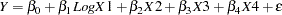where Y is the survival time, LogX1 is log(blood-clotting score), X2 is a prognostic index, X3 is an enzyme function test score, X4 is a liver function test score, andis an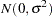error term.

A question of scientific interest is whether blood clotting score has a positive effect on survival time. Using PROC GENMOD, you can obtain a maximum likelihood estimate of the coefficient and construct a null point hypothesis to test whether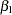is equal to 0. However, if you are interested in finding the probability that the coefficient is positive, Bayesian analysis offers a convenient alternative. You can use Bayesian analysis to directly estimate the conditional probability,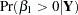, using the posterior distribution samples, which are produced as part of the output by PROC GENMOD.

The example that follows shows how to use PROC GENMOD to carry out a Bayesian analysis of the linear model with a normal error term. The SEED= option is specified to maintain reproducibility; no other options are specified in the BAYES statement. By default, a uniform prior distribution is assumed on the regression coefficients. The uniform prior is a flat prior on the real line with a distribution that reflects ignorance of the location of the parameter, placing equal likelihood on all possible values the regression coefficient can take. Using the uniform prior in the following example, you would expect the Bayesian estimates to resemble the classical results of maximizing the likelihood. If you can elicit an informative prior distribution for the regression coefficients, you should use the COEFFPRIOR= option to specify it. A default noninformative gamma prior is used for the scale parameter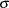.

You should make sure that the posterior distribution samples have achieved convergence before using them for Bayesian inference. PROC GENMOD produces three convergence diagnostics by default. If ODS Graphics is enabled as specified in the following SAS statements, diagnostic plots are also displayed. See the section Assessing Markov Chain Convergence for more information about convergence diagnostics and their interpretation.

Summary statistics of the posterior distribution samples are produced by default. However, these statistics might not be sufficient for carrying out your Bayesian inference, and further processing of the posterior samples might be necessary. The following SAS statements request the Bayesian analysis, and the OUTPOST= option saves the samples in the SAS data set PostSurg for further processing:

```   ods graphics on;
proc genmod data=Surg;
model y = Logx1 X2 X3 X4 / dist=normal;
bayes seed=1 OutPost=PostSurg;
run;
ods graphics off;
```

The results of this analysis are shown in the following figures.

The "Model Information" table in Figure 37.8 summarizes information about the model you fit and the size of the simulation.

Figure 37.8 Model Information
The GENMOD Procedure

Bayesian Analysis

Model Information
Data Set WORK.SURG
Burn-In Size 2000
MC Sample Size 10000
Thinning 1
Distribution Normal
Dependent Variable y Survival Time

The "Analysis of Maximum Likelihood Parameter Estimates" table in Figure 37.9 summarizes maximum likelihood estimates of the model parameters.

Figure 37.9 Maximum Likelihood Parameter Estimates
Analysis Of Maximum Likelihood Parameter Estimates
Parameter DF Estimate Standard Error Wald 95% Confidence Limits
Intercept 1 -730.559 85.4333 -898.005 -563.112
Logx1 1 171.8758 38.2250 96.9561 246.7954
x2 1 4.3019 0.5566 3.2109 5.3929
x3 1 4.0309 0.4996 3.0517 5.0100
x4 1 18.1377 12.0721 -5.5232 41.7986
Scale 1 59.8591 5.7599 49.5705 72.2832

 Note: The scale parameter was estimated by maximum likelihood.

Since no prior distributions for the regression coefficients were specified, the default noninformative uniform distributions shown in the "Uniform Prior for Regression Coefficients" table in Figure 37.10 are used. Noninformative priors are appropriate if you have no prior knowledge of the likely range of values of the parameters, and if you want to make probability statements about the parameters or functions of the parameters. See, for example, Ibrahim, Chen, and Sinha (2001) for more information about choosing prior distributions.

Figure 37.10 Regression Coefficient Priors
The GENMOD Procedure

Bayesian Analysis

Uniform Prior for Regression
Coefficients
Parameter Prior
Intercept Constant
Logx1 Constant
x2 Constant
x3 Constant
x4 Constant

The default noninformative gamma prior distribution for the normal scale parameter is shown in the "Independent Prior Distributions for Model Parameters" table in Figure 37.11.

Figure 37.11 Scale Parameter Prior
Independent Prior Distributions for
Model Parameters
Parameter Prior Distribution Hyperparameters
Shape Inverse Scale
Scale Gamma 0.001 0.001

By default, the maximum likelihood estimates of the regression parameters are used as the starting values for the simulation when noninformative prior distributions are used. These are listed in the "Initial Values and Seeds" table in Figure 37.12.

Figure 37.12 MCMC Initial Values and Seeds
Initial Values of the Chain
Chain Seed Intercept Logx1 x2 x3 x4 Scale
1 1 -730.559 171.8758 4.301896 4.030878 18.1377 59.26675

Summary statistics for the posterior sample are displayed in the "Fit Statistics," "Descriptive Statistics for the Posterior Sample," "Interval Statistics for the Posterior Sample," and "Posterior Correlation Matrix" tables in Figure 37.13, Figure 37.14, Figure 37.15, and Figure 37.16, respectively.

Figure 37.13 Fit Statistics
Fit Statistics
DIC (smaller is better) 607.406
pD (effective number of parameters) 5.912

Figure 37.14 Descriptive Statistics
The GENMOD Procedure

Bayesian Analysis

Posterior Summaries
Parameter N Mean Standard
Deviation
Percentiles
25% 50% 75%
Intercept 10000 -732.7 90.0925 -792.7 -732.2 -672.2
Logx1 10000 172.2 40.1142 145.5 171.7 198.6
x2 10000 4.3188 0.5924 3.9209 4.3166 4.7103
x3 10000 4.0477 0.5297 3.6923 4.0390 4.3966
x4 10000 17.8269 12.7611 9.3056 18.0202 26.3786
Scale 10000 63.6906 6.6360 59.0550 63.1311 67.7364

Figure 37.15 Interval Statistics
Posterior Intervals
Parameter Alpha Equal-Tail Interval HPD Interval
Intercept 0.050 -914.1 -558.4 -912.3 -557.7
Logx1 0.050 95.0527 253.0 93.5157 250.5
x2 0.050 3.1553 5.4898 3.1631 5.4928
x3 0.050 3.0250 5.1098 3.0256 5.1099
x4 0.050 -7.4580 42.6537 -7.3998 42.6693
Scale 0.050 52.3244 78.2094 51.2927 76.7926

Figure 37.16 Posterior Sample Correlation Matrix
Posterior Correlation Matrix
Parameter Intercept Logx1 x2 x3 x4 Scale
Intercept 1.000 -0.852 -0.575 -0.707 0.575 0.009
Logx1 -0.852 1.000 0.274 0.482 -0.637 -0.010
x2 -0.575 0.274 1.000 0.295 -0.473 0.004
x3 -0.707 0.482 0.295 1.000 -0.613 -0.000
x4 0.575 -0.637 -0.473 -0.613 1.000 -0.009
Scale 0.009 -0.010 0.004 -0.000 -0.009 1.000

Since noninformative prior distributions were used, the posterior sample means, standard deviations, and interval statistics shown in Figure 37.13 and Figure 37.14 are consistent with the maximum likelihood estimates shown in Figure 37.9.

By default, PROC GENMOD computes three convergence diagnostics: the lag1, lag5, lag10, and lag50 autocorrelations (Figure 37.17); Geweke diagnostic statistics (Figure 37.18); and effective sample sizes (Figure 37.19). There is no indication that the Markov chain has not converged. See the section Assessing Markov Chain Convergence for more information about convergence diagnostics and their interpretation.

Figure 37.17 Posterior Sample Autocorrelations
The GENMOD Procedure

Bayesian Analysis

Posterior Autocorrelations
Parameter Lag 1 Lag 5 Lag 10 Lag 50
Intercept 0.4525 0.0478 0.0104 -0.0083
Logx1 0.4226 0.0426 0.0078 -0.0052
x2 0.2328 0.0307 0.0123 -0.0057
x3 0.4020 0.0501 0.0009 -0.0034
x4 0.5906 0.0767 0.0124 0.0026
Scale 0.0941 0.0116 -0.0113 0.0050

Figure 37.18 Geweke Diagnostic Statistics
Geweke Diagnostics
Parameter z Pr > |z|
Intercept -1.0049 0.3149
Logx1 1.2048 0.2283
x2 0.4330 0.6650
x3 0.8662 0.3864
x4 -0.9039 0.3661
Scale -1.0094 0.3128

Figure 37.19 Effective Sample Sizes
Effective Sample Sizes
Parameter ESS Correlation
Time
Efficiency
Intercept 3083.6 3.2430 0.3084
Logx1 3185.9 3.1389 0.3186
x2 5051.4 1.9797 0.5051
x3 3281.7 3.0472 0.3282
x4 2534.6 3.9454 0.2535
Scale 8107.8 1.2334 0.8108

Trace, autocorrelation, and density plots for the seven model parameters, shown in Figure 37.20 through Figure 37.25, are useful in diagnosing whether the Markov chain of posterior samples has converged. These plots show no evidence that the chain has not converged. See the section Visual Analysis via Trace Plots for help with interpreting these diagnostic plots.

Figure 37.20 Diagnostic Plots for Intercept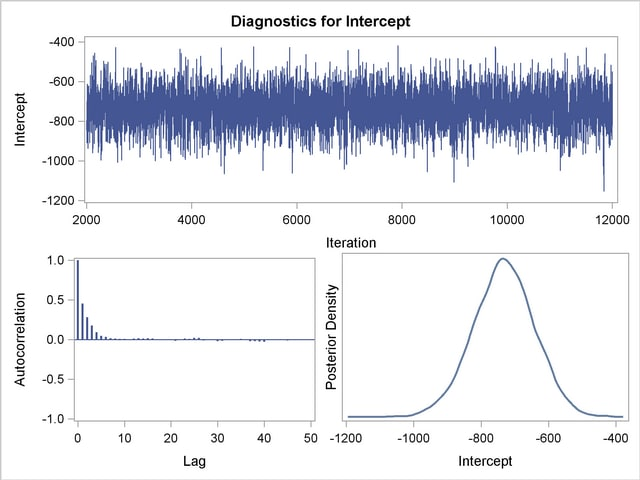Figure 37.21 Diagnostic Plots for logX1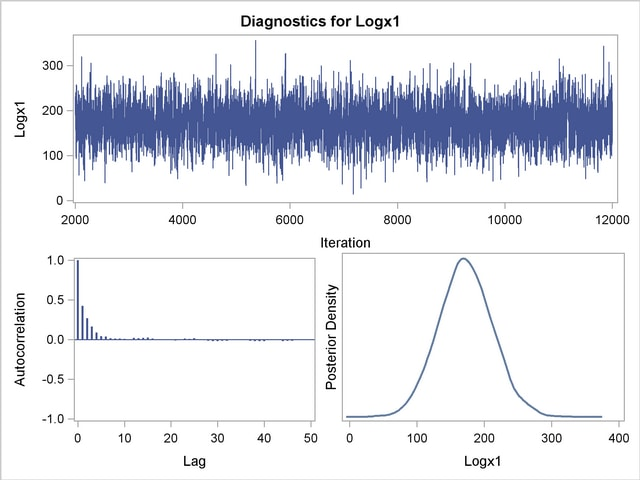Figure 37.22 Diagnostic Plots for X2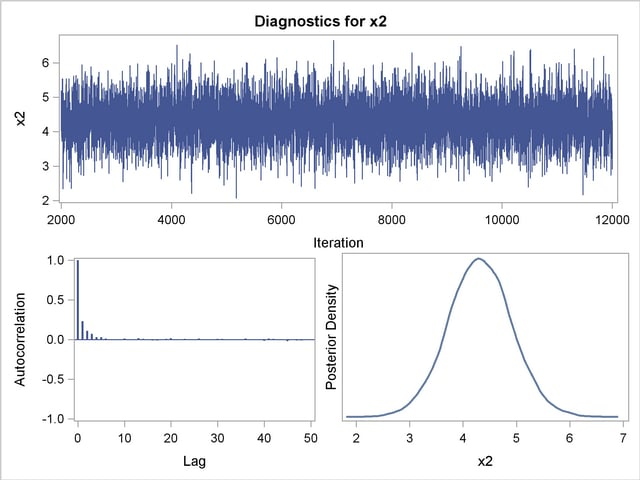Figure 37.23 Diagnostic Plots for X3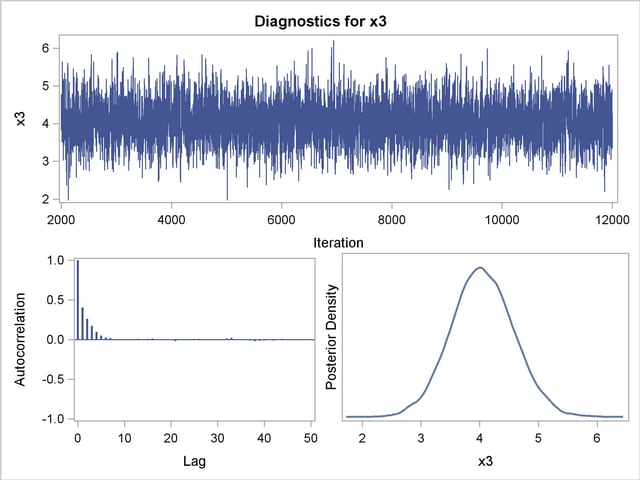Figure 37.24 Diagnostic Plots for X4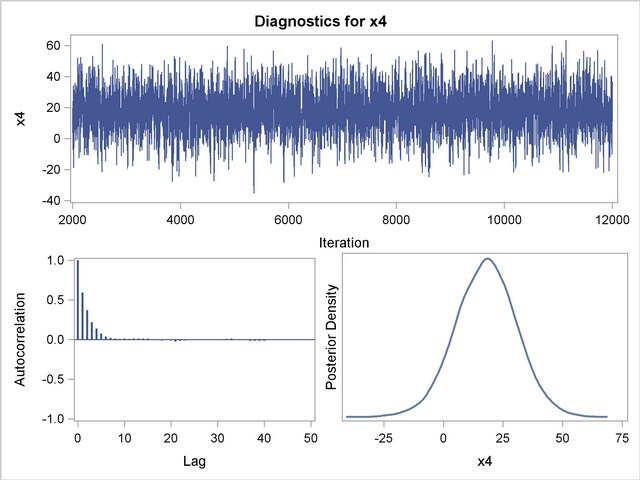Figure 37.25 Diagnostic Plots for X5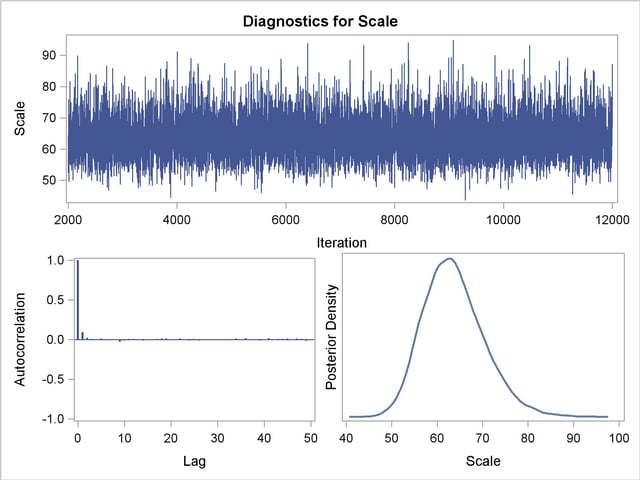Suppose, for illustration, a question of scientific interest is whether blood clotting score has a positive effect on survival time. Since the model parameters are regarded as random quantities in a Bayesian analysis, you can answer this question by estimating the conditional probability ofbeing positive, given the data,, from the posterior distribution samples. The following SAS statements compute the estimate of the probability ofbeing positive:

```   data Prob;
set PostSurg;
Indicator = (logX1 > 0);
label Indicator= 'log(Blood Clotting Score) > 0';
run;

proc Means data = Prob(keep=Indicator) n mean;
run;
```

As shown in Figure 37.26, there is a 1.00 probability of a positive relationship between the logarithm of a blood clotting score and survival time, adjusted for the other covariates.

Figure 37.26 Probability That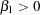The MEANS Procedure

Analysis Variable : Indicator
log(Blood Clotting Score)
> 0
N Mean
10000 1.0000000Previous Page | Next Page | Top of Page